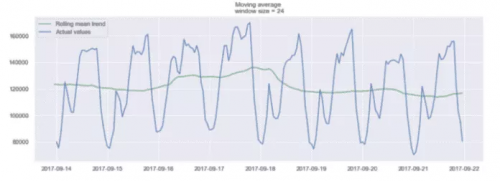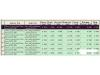# 时序分析有哪些乐趣？如何才能精准预测？

2019-08-16 10:27:39 来源： AI开发者ARIMA24 小时窗口上的移动平均值示例12 小时窗口上的移动平均值示例SARIMA 实际上是简单模型的组合，可以生成一个复杂的模型，该模型可以模拟具有非平稳特性和季节性的时间序列。import numpy as np
import pandas as pd
import matplotlib.pyplot as plt
import seaborn as sns
sns.set()

from sklearn.metrics import r2_score, median_absolute_error, mean_absolute_error
from sklearn.metrics import median_absolute_error, mean_squared_error, mean_squared_log_error

from scipy.optimize import minimize
import statsmodels.tsa.api as smt
import statsmodels.api as sm

from tqdm import tqdm_notebook

from itertools import product

def mean_absolute_percentage_error(y_true, y_pred):
return np.mean(np.abs((y_true - y_pred) / y_true)) * 100

import warnings
warnings.filterwarnings('ignore')

%matplotlib inline

DATAPATH = 'data/stock_prices_sample.csv'

data = pd.read_csv(DATAPATH, index_col=['DATE'], parse_dates=['DATE'])data = data[data.TICKER != 'GEF']
data = data[data.TYPE != 'Intraday']

data.drop(drop_cols, axis=1, inplace=True)# Plot closing price

plt.figure(figsize=(17, 8))
plt.plot(data.CLOSE)
plt.title('Closing price of New Germany Fund Inc (GF)')
plt.ylabel('Closing price (\$)')
plt.grid(False)
plt.show()New Germany Fund （GF）收盘价

def plot_moving_average(series, window, plot_intervals=False, scale=1.96):

rolling_mean = series.rolling(window=window).mean()

plt.figure(figsize=(17,8))
plt.title('Moving average\n window size = {}'.format(window))
plt.plot(rolling_mean, 'g', label='Rolling mean trend')

#Plot confidence intervals for smoothed values
if plot_intervals:
mae = mean_absolute_error(series[window:], rolling_mean[window:])
deviation = np.std(series[window:] - rolling_mean[window:])
lower_bound = rolling_mean - (mae + scale * deviation)
upper_bound = rolling_mean + (mae + scale * deviation)
plt.plot(upper_bound, 'r--', label='Upper bound / Lower bound')
plt.plot(lower_bound, 'r--')

plt.plot(series[window:], label='Actual values')
plt.legend(loc='best')
plt.grid(True)

#Smooth by the previous 5 days (by week)
plot_moving_average(data.CLOSE, 5)

#Smooth by the previous month (30 days)
plot_moving_average(data.CLOSE, 30)

#Smooth by previous quarter (90 days)
plot_moving_average(data.CLOSE, 90, plot_intervals=True)def exponential_smoothing(series, alpha):

result = [series] # first value is same as series
for n in range(1, len(series)):
result.append(alpha * series[n] + (1 - alpha) * result[n-1])
return result

def plot_exponential_smoothing(series, alphas):

plt.figure(figsize=(17, 8))
for alpha in alphas:
plt.plot(exponential_smoothing(series, alpha), label="Alpha {}".format(alpha))
plt.plot(series.values, "c", label = "Actual")
plt.legend(loc="best")
plt.axis('tight')
plt.title("Exponential Smoothing")
plt.grid(True);

plot_exponential_smoothing(data.CLOSE, [0.05, 0.3])

def double_exponential_smoothing(series, alpha, beta):

result = [series]
for n in range(1, len(series)+1):
if n == 1:
level, trend = series, series - series
if n >= len(series): # forecasting
value = result[-1]
else:
value = series[n]
last_level, level = level, alpha * value + (1 - alpha) * (level + trend)
trend = beta * (level - last_level) + (1 - beta) * trend
result.append(level + trend)
return result

def plot_double_exponential_smoothing(series, alphas, betas):

plt.figure(figsize=(17, 8))
for alpha in alphas:
for beta in betas:
plt.plot(double_exponential_smoothing(series, alpha, beta), label="Alpha {}, beta {}".format(alpha, beta))
plt.plot(series.values, label = "Actual")
plt.legend(loc="best")
plt.axis('tight')
plt.title("Double Exponential Smoothing")
plt.grid(True)

plot_double_exponential_smoothing(data.CLOSE, alphas=[0.9, 0.02], betas=[0.9, 0.02])def tsplot(y, lags=None, figsize=(12, 7), syle='bmh'):

if not isinstance(y, pd.Series):
y = pd.Series(y)

with plt.style.context(style='bmh'):
fig = plt.figure(figsize=figsize)
layout = (2,2)
ts_ax = plt.subplot2grid(layout, (0,0), colspan=2)
acf_ax = plt.subplot2grid(layout, (1,0))
pacf_ax = plt.subplot2grid(layout, (1,1))

y.plot(ax=ts_ax)
ts_ax.set_title('Time Series Analysis Plots\n Dickey-Fuller: p={0:.5f}'.format(p_value))
smt.graphics.plot_acf(y, lags=lags, ax=acf_ax)
smt.graphics.plot_pacf(y, lags=lags, ax=pacf_ax)
plt.tight_layout()

tsplot(data.CLOSE, lags=30)

# Take the first difference to remove to make the process stationary
data_diff = data.CLOSE - data.CLOSE.shift(1)

tsplot(data_diff[1:], lags=30)SARIMA
#Set initial values and some bounds
ps = range(0, 5)
d = 1
qs = range(0, 5)
Ps = range(0, 5)
D = 1
Qs = range(0, 5)
s = 5

#Create a list with all possible combinations of parameters
parameters = product(ps, qs, Ps, Qs)
parameters_list = list(parameters)
len(parameters_list)

# Train many SARIMA models to find the best set of parameters
def optimize_SARIMA(parameters_list, d, D, s):
"""
Return dataframe with parameters and corresponding AIC

parameters_list - list with (p, q, P, Q) tuples
d - integration order
D - seasonal integration order
s - length of season
"""

results = []
best_aic = float('inf')

for param in tqdm_notebook(parameters_list):
try: model = sm.tsa.statespace.SARIMAX(data.CLOSE, order=(param, d, param),
seasonal_order=(param, D, param, s)).fit(disp=-1)
except:
continue

aic = model.aic

#Save best model, AIC and parameters
if aic < best_aic:
best_model = model
best_aic = aic
best_param = param
results.append([param, model.aic])

result_table = pd.DataFrame(results)
result_table.columns = ['parameters', 'aic']
#Sort in ascending order, lower AIC is better
result_table = result_table.sort_values(by='aic', ascending=True).reset_index(drop=True)

return result_table

result_table = optimize_SARIMA(parameters_list, d, D, s)

#Set parameters that give the lowest AIC (Akaike Information Criteria)
p, q, P, Q = result_table.parameters

best_model = sm.tsa.statespace.SARIMAX(data.CLOSE, order=(p, d, q),
seasonal_order=(P, D, Q, s)).fit(disp=-1)

print(best_model.summary())# Make a dataframe containing actual and predicted prices
comparison = pd.DataFrame({'actual': [18.93, 19.23, 19.08, 19.17, 19.11, 19.12],
'predicted': [18.96, 18.97, 18.96, 18.92, 18.94, 18.92]},
index = pd.date_range(start='2018-06-05', periods=6,))

#Plot predicted vs actual price

plt.figure(figsize=(17, 8))
plt.plot(comparison.actual)
plt.plot(comparison.predicted)
plt.title('Predicted closing price of New Germany Fund Inc (GF)')
plt.ylabel('Closing price (\$)')
plt.legend(loc='best')
plt.grid(False)
plt.show()import warnings
warnings.filterwarnings('ignore')

import numpy as np
import pandas as pd
from scipy import stats
import statsmodels.api as sm
import matplotlib.pyplot as plt

%matplotlib inline

DATAPATH = 'data/AirQualityUCI.csv'

data = pd.read_csv(DATAPATH, sep=';')# Make dates actual dates
data['Date'] = pd.to_datetime(data['Date'])

# Convert measurements to floats
for col in data.iloc[:,2:].columns:
if data[col].dtypes == object:
data[col] = data[col].str.replace(',', '.').astype('float')

# Compute the average considering only the positive values
def positive_average(num):
return num[num > -200].mean()

# Aggregate data
daily_data = data.drop('Time', axis=1).groupby('Date').apply(positive_average)

# Drop columns with more than 8 NaN
daily_data = daily_data.iloc[:,(daily_data.isna().sum() <= 8).values]

# Remove rows containing NaN values
daily_data = daily_data.dropna()

# Aggregate data by week
weekly_data = daily_data.resample('W').mean()

# Plot the weekly concentration of each gas
def plot_data(col):
plt.figure(figsize=(17, 8))
plt.plot(weekly_data[col])
plt.xlabel('Time')
plt.ylabel(col)
plt.grid(False)
plt.show()

for col in weekly_data.columns:
plot_data(col)NOx 浓度

# Drop irrelevant columns
cols_to_drop = ['PT08.S1(CO)', 'C6H6(GT)', 'PT08.S2(NMHC)', 'PT08.S4(NO2)', 'PT08.S5(O3)', 'T', 'RH', 'AH']

weekly_data = weekly_data.drop(cols_to_drop, axis=1)

# Import Prophet
from fbprophet import Prophet
import logging

logging.getLogger().setLevel(logging.ERROR)

# Change the column names according to Prophet's guidelines
df = weekly_data.reset_index()
df.columns = ['ds', 'y']

# Split into a train/test set
prediction_size = 30
train_df = df[:-prediction_size]

# Initialize and train a model
m = Prophet()
m.fit(train_df)

# Make predictions
future = m.make_future_dataframe(periods=prediction_size)
forecast = m.predict(future)

# Plot forecast
m.plot(forecast)

# Plot forecast's components
m.plot_components(forecast)

# Evaluate the model
def make_comparison_dataframe(historical, forecast):
return forecast.set_index('ds')[['yhat', 'yhat_lower', 'yhat_upper']].join(historical.set_index('ds'))

cmp_df = make_comparison_dataframe(df, forecast)

def calculate_forecast_errors(df, prediction_size):

df = df.copy()

df['e'] = df['y'] - df['yhat']
df['p'] = 100 * df['e'] / df['y']

predicted_part = df[-prediction_size:]

error_mean = lambda error_name: np.mean(np.abs(predicted_part[error_name]))

return {'MAPE': error_mean('p'), 'MAE': error_mean('e')}

for err_name, err_value in calculate_forecast_errors(cmp_df, prediction_size).items():
print(err_name, err_value)

# Plot forecast with upper and lower bounds
plt.figure(figsize=(17, 8))
plt.plot(cmp_df['yhat'])
plt.plot(cmp_df['yhat_lower'])
plt.plot(cmp_df['yhat_upper'])
plt.plot(cmp_df['y'])
plt.xlabel('Time')
plt.ylabel('Average Weekly NOx Concentration')
plt.grid(False)
plt.show()

Prophet 要求日期列命名为 ds，特征列命名为 y，因此我们进行了适当的更改。Prophet 让你可以轻松绘制预测图，我们得到：

NOx 浓度预测via：https://towardsdatascience.com/the-complete-guide-to-time-series-analysis-and-forecasting-70d476bfe775FPGA（Field-Programmable Gate Array），即现场可编程门阵列，它是作为专用集成电路（ASIC）领域中的一种半定制电路而出现的，既解决了定制电路的不足，又克服了原有可编程器件门电路数有限的缺点。对于时序如何用FPGA来分析与设计，本文将详细介绍。

Viavi Solutions公司（纳斯达克股票代码：VIAV）今日宣布推出全新Network & Service Companion（NSC）系列外场测试仪器中的首款产品NSC-100。

ST专区